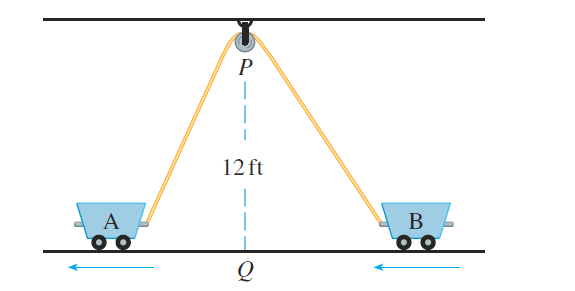#### Problem 42E

42. Two carts, $A$ and $B$, are connected by a rope $39 \mathrm{ft}$ long that passes over a pulley $P$ (see the figure). The point $Q$ is on the floor $12 \mathrm{ft}$ directly beneath $P$ and between the carts. Cart $\mathrm{A}$ is being pulled away from $Q$ at a speed of $2 \mathrm{ft} / \mathrm{s}$. How fast is cart B moving toward $Q$ at the instant when cart $\mathrm{A}$ is $5 \mathrm{ft}$ from $Q$ ?